5-Color Theorem

5-color theorem – Every planar graph is 5-colorable.

Proof:

Let G be the smallest planar graph (in terms of number of vertices) that cannot be colored with five colors.

Let v be a vertex in G that has the maximum degree.  We know that deg(v) < 6 (from the corollary to Euler’s formula).

Case #1: deg(v) ≤ 4.  G-v can be colored with five colors.

There are at most 4 colors that have been used on the neighbors of v.  There is at least one color then available for v.

So G can be colored with five colors, a contradiction.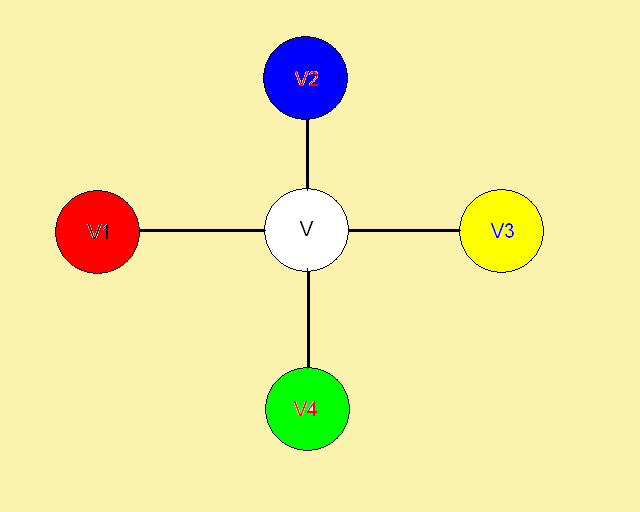Case #2: deg(v) = 5.  G-v can be colored with 5 colors.

If two of the neighbors of v are colored with the same color, then there is a color available for v.

So we may assume that all the vertices that are adjacent to v are colored with colors 1,2,3,4,5 in the clockwise order.

Consider all the vertices being colored with colors 1 and 3 (and all the edges among them).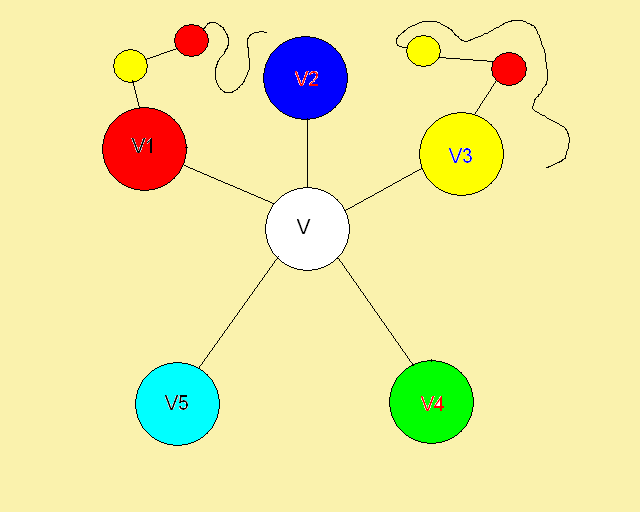If this subgraph G is disconnected and v1 and v3 are in different components, then we can switch the colors 1 and 3 in the component with v1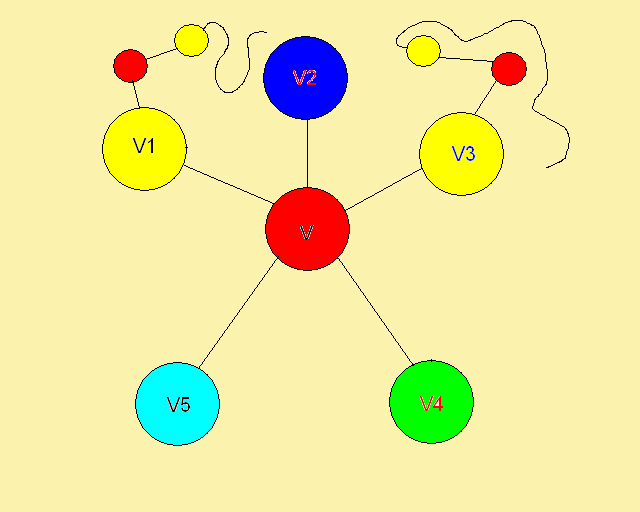This will still be a 5-coloring of G-v.  Furthermore, v1 is colored with color 3 in this new 5-coloring and v3 is still colored with color 3.  Color 1 would be available for v, a contradiction.

Therefore v1 and v3 must be in the same component in that subgraph, i.e. there is a path from v1 to v3 such that every vertex on this path is colored with either color 1 or color 3.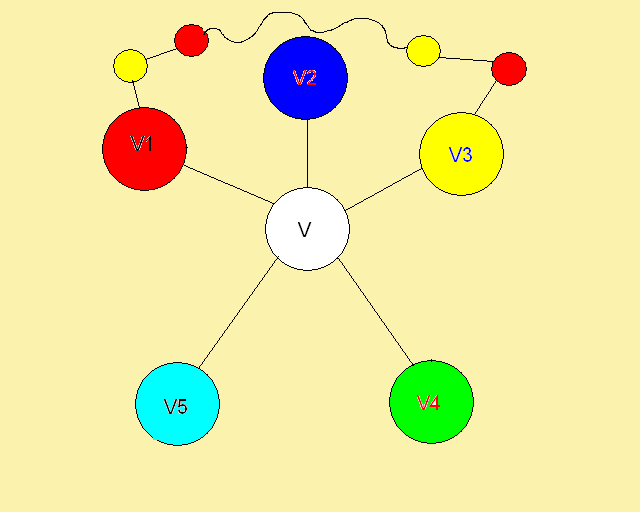Now, consider all the vertices being colored with colors 2 and 4 (and all the edges among them).   If v2 and v4 don't lie of the same connected component then we can interchange the colors in the chain starting at v2 and use left over color for v.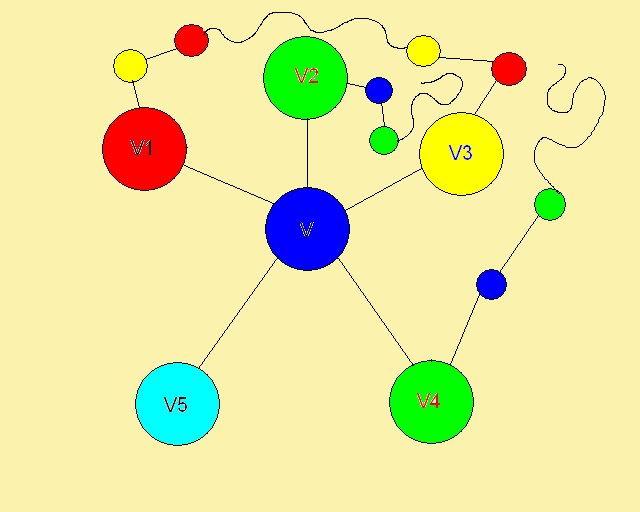If they do lie on the same connected component then there is a path from v2 to v4 such that every vertex on that path has either color 2 or color 4.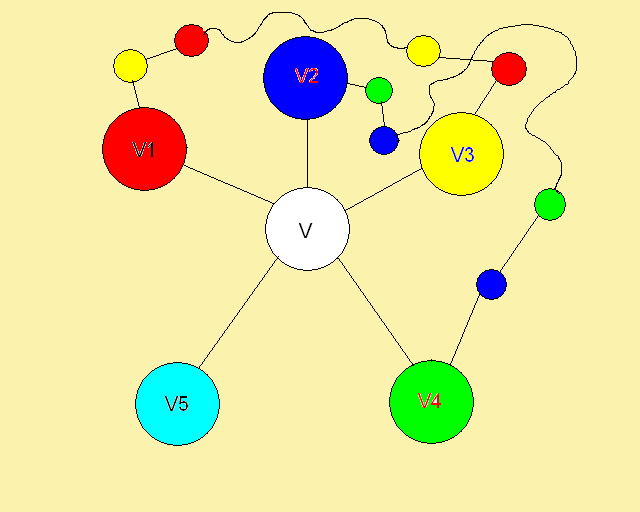This means that there must be two edges that cross each other.  This contradicts the planarity of the graph and hence concludes the proof. ڤ

PREVIOUS: Theorems    NEXT: Algorithm